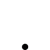# 針對每根1分K設定指標的值

•   33
• 最後發表   CH____________  2 週前
CH____________ 發文於   2021/10/07

Hello 小幫手你好,因為我有用python算一些資料,想匯入到XQ中變成指標 輔助研究

if time==09:00 Plot1(10, "強度");

if time==09:01 Plot2(30"強度");

if time==09:02 Plot3(-50"強度");

XQ小幫手 發文於   2021/10/14

Hello CH____________,

XQ無法匯入外部的資訊。

if date = 20211013 and time = 090000 then value1 = 10

else if date = 20211013 and time = 090100 then value1 = 30

else if ......

plot1(value1, "強度");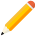發表新主題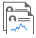我的發文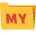我的收藏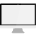分類

Close